Vectors - Motion and Forces in Two Dimensions - Lesson 3 - Forces in Two Dimensions

# Equilibrium and Statics

When all the forces that act upon an object are balanced, then the object is said to be in a state of equilibrium. The forces are considered to be balanced if the rightward forces are balanced by the leftward forces and the upward forces are balanced by the downward forces. This however does not necessarily mean that all the forces are equal to each other. Consider the two objects pictured in the force diagram shown below. Note that the two objects are at equilibrium because the forces that act upon them are balanced; however, the individual forces are not equal to each other. The 50 N force is not equal to the 30 N force.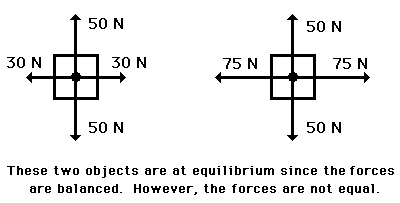If an object is at equilibrium, then the forces are balanced. Balanced is the key word that is used to describe equilibrium situations. Thus, the net force is zero and the acceleration is 0 m/s/s. Objects at equilibrium must have an acceleration of 0 m/s/s. This extends from Newton's first law of motion. But having an acceleration of 0 m/s/s does not mean the object is at rest. An object at equilibrium is either ...

• at rest and staying at rest, or
• in motion and continuing in motion with the same speed and direction.

This too extends from Newton's first law of motion.

### Analyzing a Static Equilibrium Situation

If an object is at rest and is in a state of equilibrium, then we would say that the object is at "static equilibrium." "Static" means stationary or at rest. A common physics lab is to hang an object by two or more strings and to measure the forces that are exerted at angles upon the object to support its weight. The state of the object is analyzed in terms of the forces acting upon the object. The object is a point on a string upon which three forces were acting. See diagram at right. If the object is at equilibrium, then the net force acting upon the object should be 0 Newton. Thus, if all the forces are added together as vectors, then the resultant force (the vector sum) should be 0 Newton. (Recall that the net force is "the vector sum of all the forces" or the resultant of adding all the individual forces head-to-tail.) Thus, an accurately drawn vector addition diagram can be constructed to determine the resultant. Sample data for such a lab are shown below.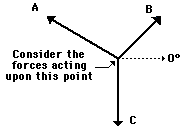Force A Force B Force C Magnitude 3.4 N 9.2 N 9.8 N Direction 161 deg. 70 deg. 270 deg

For most students, the resultant was 0 Newton (or at least very close to 0 N). This is what we expected - since the object was at equilibrium, the net force (vector sum of all the forces) should be 0 N.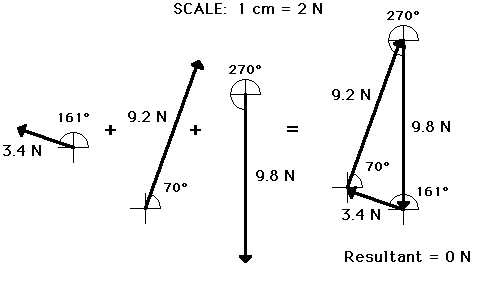Another way of determining the net force (vector sum of all the forces) involves using the trigonometric functions to resolve each force into its horizontal and vertical components. Once the components are known, they can be compared to see if the vertical forces are balanced and if the horizontal forces are balanced. The diagram below shows vectors A, B, and C and their respective components. For vectors A and B, the vertical components can be determined using the sine of the angle and the horizontal components can be analyzed using the cosine of the angle. The magnitude and direction of each component for the sample data are shown in the table below the diagram.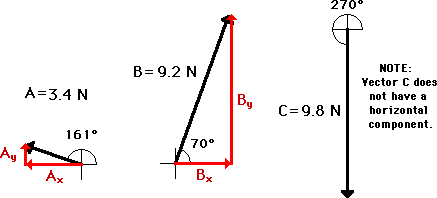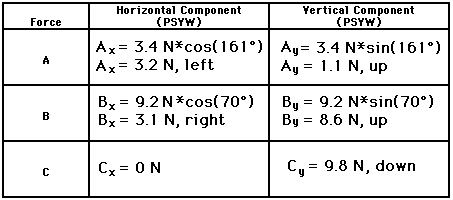The data in the table above show that the forces nearly balance. An analysis of the horizontal components shows that the leftward component of A nearly balances the rightward component of B. An analysis of the vertical components show that the sum of the upward components of A + B nearly balance the downward component of C. The vector sum of all the forces is (nearly) equal to 0 Newton. But what about the 0.1 N difference between rightward and leftward forces and the 0.2 N difference between the upward and downward forces? Why do the components of force only nearly balance? The sample data used in this analysis are the result of measured data from an actual experimental setup. The difference between the actual results and the expected results is due to the error incurred when measuring force A and force B. We would have to conclude that this low margin of experimental error reflects an experiment with excellent results. We could say it's "close enough for government work."

###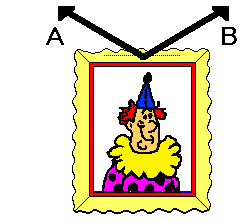Analyzing a Hanging Sign

The above analysis of the forces acting upon an object in equilibrium is commonly used to analyze situations involving objects at static equilibrium. The most common application involves the analysis of the forces acting upon a sign that is at rest. For example, consider the picture at the right that hangs on a wall. The picture is in a state of equilibrium, and thus all the forces acting upon the picture must be balanced. That is, all horizontal components must add to 0 Newton and all vertical components must add to 0 Newton. The leftward pull of cable A must balance the rightward pull of cable B and the sum of the upward pull of cable A and cable B must balance the weight of the sign.

Suppose the tension in both of the cables is measured to be 50 N and that the angle that each cable makes with the horizontal is known to be 30 degrees. What is the weight of the sign? This question can be answered by conducting a force analysis using trigonometric functions. The weight of the sign is equal to the sum of the upward components of the tension in the two cables. Thus, a trigonometric function can be used to determine this vertical component. A diagram and accompanying work is shown below.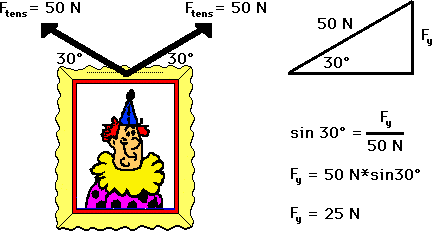Since each cable pulls upwards with a force of 25 N, the total upward pull of the sign is 50 N. Therefore, the force of gravity (also known as weight) is 50 N, down. The sign weighs 50 N.

In the above problem, the tension in the cable and the angle that the cable makes with the horizontal are used to determine the weight of the sign. The idea is that the tension, the angle, and the weight are related. If the any two of these three are known, then the third quantity can be determined using trigonometric functions.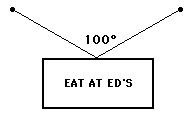As another example that illustrates this idea, consider the symmetrical hanging of a sign as shown at the right. If the sign is known to have a mass of 5 kg and if the angle between the two cables is 100 degrees, then the tension in the cable can be determined. Assuming that the sign is at equilibrium (a good assumption if it is remaining at rest), the two cables must supply enough upward force to balance the downward force of gravity. The force of gravity (also known as weight) is 49 N (Fgrav = m*g), so each of the two cables must pull upwards with 24.5 N of force. Since the angle between the cables is 100 degrees, then each cable must make a 50-degree angle with the vertical and a 40-degree angle with the horizontal. A sketch of this situation (see diagram below) reveals that the tension in the cable can be found using the sine function. The triangle below illustrates these relationships.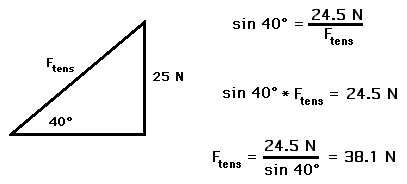### Thinking Conceptually

There is an important principle that emanates from some of the trigonometric calculations performed above. The principle is that as the angle with the horizontal increases, the amount of tensional force required to hold the sign at equilibrium decreases. To illustrate this, consider a 10-Newton picture held by three different wire orientations as shown in the diagrams below. In each case, two wires are used to support the picture; each wire must support one-half of the sign's weight (5 N). The angle that the wires make with the horizontal is varied from 60 degrees to 15 degrees. Use this information and the diagram below to determine the tension in the wire for each orientation. When finished, click the button to view the answers.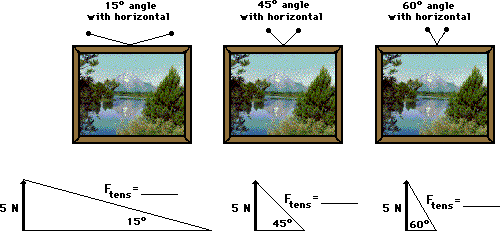In conclusion, equilibrium is the state of an object in which all the forces acting upon it are balanced. In such cases, the net force is 0 Newton. Knowing the forces acting upon an object, trigonometric functions can be utilized to determine the horizontal and vertical components of each force. If at equilibrium, then all the vertical components must balance and all the horizontal components must balance.

### We Would Like to Suggest ...Sometimes it isn't enough to just read about it. You have to interact with it! And that's exactly what you do when you use one of The Physics Classroom's Interactives. We would like to suggest that you combine the reading of this page with the use of our Name That Vector Interactive, our Vector Addition Interactive, or our Vector Guessing Game Interactive. All three Interactives can be found in the Physics Interactive section of our website and provide an interactive experience with the skill of adding vectors.

### Check Your Understanding

The following questions are meant to test your understanding of equilibrium situations. Click the button to view the answers to these questions.

1. The following picture is hanging on a wall. Use trigonometric functions to determine the weight of the picture.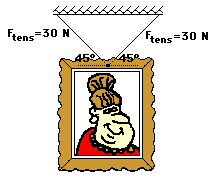2. The sign below hangs outside the physics classroom, advertising the most important truth to be found inside. The sign is supported by a diagonal cable and a rigid horizontal bar. If the sign has a mass of 50 kg, then determine the tension in the diagonal cable that supports its weight.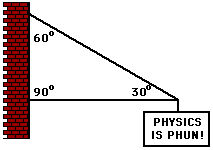3. The following sign can be found in Glenview. The sign has a mass of 50 kg. Determine the tension in the cables.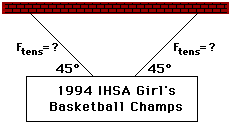4. After its most recent delivery, the infamous stork announces the good news. If the sign has a mass of 10 kg, then what is the tensional force in each cable? Use trigonometric functions and a sketch to assist in the solution.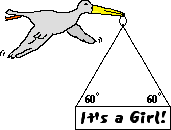5. Suppose that a student pulls with two large forces (F1 and F2) in order to lift a 1-kg book by two cables. If the cables make a 1-degree angle with the horizontal, then what is the tension in the cable?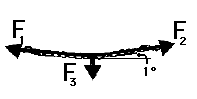Next Section:

Follow Us